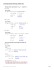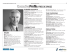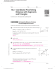# Solve the problems below to answer the riddle:

## Transcription

Solve the problems below to answer the riddle:
```Name
1-3
Class
Date
Puzzle: What Is a Line’s Favorite Kind of Fruit?
Measuring Segments
Solve the problems below to answer the
riddle:
What is a line’s favorite kind of fruit?
right and put the corresponding letter in
the answer blank at the bottom of the page.
1. Points A, B, and C are collinear and A is between B and C.
AB = 4x − 3, BC = 7x + 5, and AC = 5x − 16.
Find each value.
a. BC
b. AB
c. AC
2. On a number line, G = 8 and H = –3. If H is the midpoint
of GI , find the coordinate of I.
3. J is the midpoint of KL . Find KJ if KL = 38.
For 4–6, refer to the number line below.
4. Suppose O is the midpoint of MQ and N is the midpoint of
MO . If NO = 8, find MQ.
5. Suppose P is the midpoint of NQ , OP = 11, and OQ = 35.
Find NO.
6. If NO = 2y + 11, OP = 3y − 2, NP = 6y + 3, and
MP = 64, find each value.
a. NO
b. MN
Prentice Hall Geometry • Activities, Games, and Puzzles
3
```

### The Midpoint Formula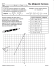### Algebra with shapes How to . . .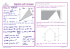### 1-2 Practice A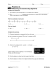### Guidelines TV Lab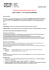### Reteach Sec 1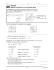### Coordinate Geometry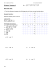### Lesson 30: Special Lines in Triangles M1 Lesson 30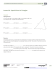### 5.1 Midsegment Theorem and Coordinate Proof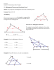### STUDY GUIDE for Unit 1 Test Geometry 1. Z?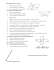### Time: 3 to 3 ½ hours M.M.:90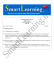### C Geometry Name: 1.4 Worksheet – Constructing Parallel and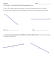### BONSAI WG on Global Impact Assessment (GIA)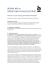### HSM12CC_GM_06_09_CM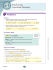### Mrs. Turkus - Littleton Public Schools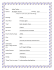### Speed-Dating Questions - Be the Change Consulting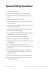### Casa de Fruta Celebrating 100 Years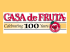### Babich Wines Limited - East Coast Vintara Red 2012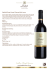### Pro(fessional) Seminar 2 EDCI 888 4/4/12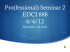### 2. SOLUTION - Waynesville R